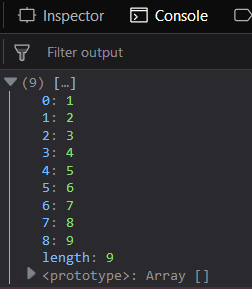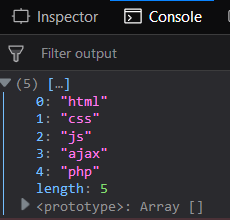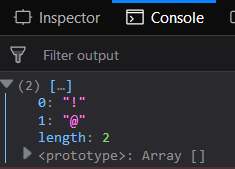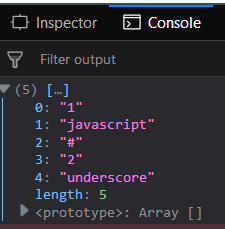Open In App
Related Articles

# Underscore.js _.initial() Function

The Underscore.js is a JavaScript library that provides a lot of useful functions like the map, filter, invoke etc even without using any built-in objects.
The _.initial() function is used to exclude the last element from the array. This function is used to perform some action on all the elements except last element. This is a basic function which helps to differentiate between the last element of the array and all the rest elements.
Syntax:

`_.initial( array, [n] ) `

Parameters: This function accepts two parameter which are listed below:

• array: This parameter is used to hold the array elements.
• n: This parameter is used to hold the last element n.

Return value: It returns an array except the last element.
Passing a list of numbers to _.initial() function: The ._initial() function takes the element from the list one by one and then add this element to the resultant array. It is used to print all the elements except the last element.
Example:

## html

 ```<``html``>``    ``<``head``>``        ``<``script` `src =``"https://cdnjs.cloudflare.com/ajax/libs/underscore.js/1.9.1/underscore-min.js" >``        ````    ````    ``<``body``>``        ``<``script` `type="text/javascript">``            ``console.log(_.initial([1, 2, 3, 4, 5,``                            ``6, 7, 8, 9, 10]));``        ````    ````                   `

Output:Passing words to the _.initial() function: The ._initial() function takes the element which is here alphabets from the list one by one and then add this element to the resultant array. The resultant array also gives the length of the resultant array. Then console.log() the resultant array.
Example:

## html

 ```<``html``>``    ``<``head``>``        ``<``script` `src =``"https://cdnjs.cloudflare.com/ajax/libs/underscore.js/1.9.1/underscore-min.js" >``        ````    ````    ``<``body``>``        ``<``script` `type="text/javascript">``            ``console.log(_.initial(['html', 'css', 'js',``                            ``'ajax', 'php', 'node.js']));``        ````    ````                   `

Output:Using the second parameter of the _.initial() function: The second parameter is used to select the elements from the array except the number of elements which is given in second parameter and display them. The second parameter is very crucial to display all the elements except the number given in the second parameter from the right side in the array passed.
Example:

## html

 ```<``html``>``    ``<``head``>``        ``<``script` `src =``"https://cdnjs.cloudflare.com/ajax/libs/underscore.js/1.9.1/underscore-min.js" >``        ````    ````    ``<``body``>``        ``<``script` `type="text/javascript">``            ``console.log(_.initial(['!', '@', '#',``                            ``'\$', '%', '^']), 4);``        ````    ````                   `

Output:Using both the words and the numbers in the _.initial() function: It takes the elements from the zeroth index of the given array and displays all the element. The fact that the array elements are words, alphabets or the numbers is not important. The last element is not included in the list.
Example:

## html

 ```<``html``>``    ``<``head``>``        ``<``script` `src =``"https://cdnjs.cloudflare.com/ajax/libs/underscore.js/1.9.1/underscore-min.js" >``        ````    ````    ``<``body``>``        ``<``script` `type="text/javascript">``            ``console.log(_.initial(['1', 'javascript',``                        ``'#', '2', 'underscore', '^']));``        ````    ````                   `

Output:Note: These commands will not work in Google console or in Firefox as for these additional files need to be added which they didn’t have added. So, add the given links to your HTML file and then run them.

## html

 `<``script` `type="text/javascript" src =``"https://cdnjs.cloudflare.com/ajax/libs/underscore.js/1.9.1/underscore-min.js">```